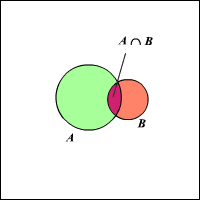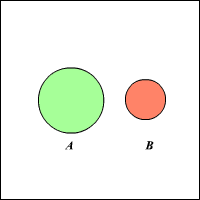If $A$ and $B$ are two events in a probability experiment, then the probability that either one of the events will occur is:

This can be represented in a Venn diagram as:

$P\left(A\cup B\right)=P\left(A\right)+P\left(B\right)-P\left(A\cap B\right)$If $A$ and $B$ are two mutually exclusive events , $P\left(A\cap B\right)=0$ . Then the probability that either one of the events will occur is:

This can be represented in a Venn diagram as:

$P\left(A\cup B\right)=P\left(A\right)+P\left(B\right)$Example:

If you take out a single card from a regular pack of cards, what is probability that the card is either an ace or spade?

Let $X$ be the event of picking an ace and $Y$ be the event of picking a spade.

$P\left(X\right)=\frac{4}{52}$

$P\left(Y\right)=\frac{13}{52}$

The two events are not mutually exclusive, as there is one favorable outcome in which the card can be both an ace and spade.